• matlab多个excel读取并存到一个excel的不同sheet
• 有很新手小白会被Matlab如何读取excel文件里数据的问题困惑住，所以小编就带来了Matlab读取excel文件里数据的操作方法，感兴趣的朋友就跟小编一起来学习一下吧。Matlab读取excel文件里数据的操作方法以03版excel为...

有很多新手小白会被Matlab如何读取excel文件里数据的问题困惑住，所以小编就带来了Matlab读取excel文件里数据的操作方法，感兴趣的朋友就跟小编一起来学习一下吧。
Matlab读取excel文件里数据的操作方法以03版excel为例，假设excel中数据为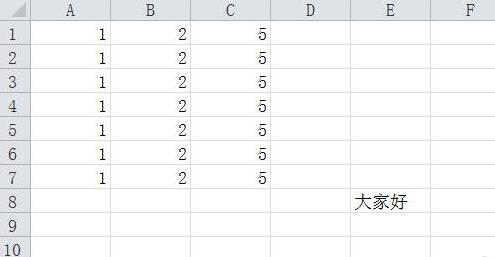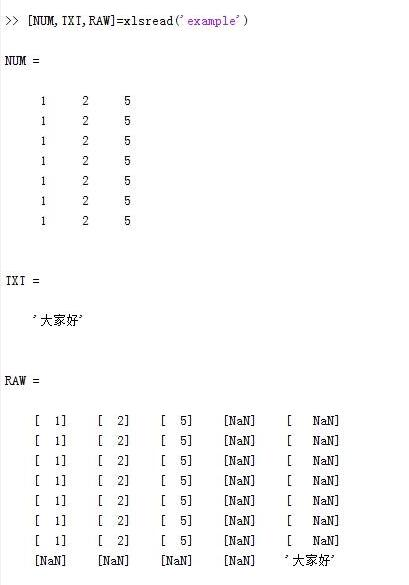读取指定sheet中的数据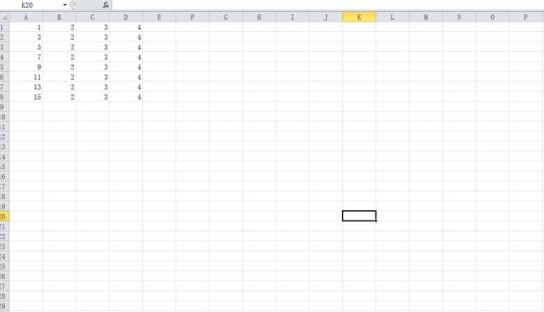结果如下：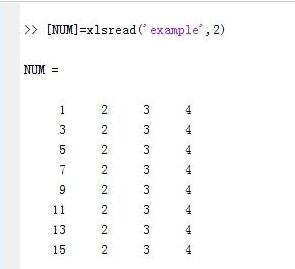读取指定单元格中的数据
以sheet2中的数据为例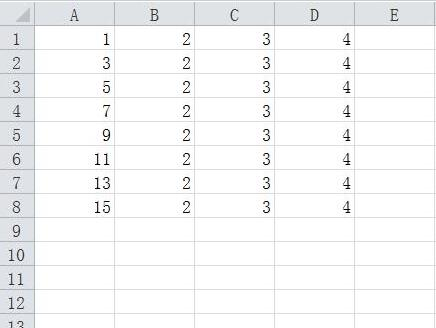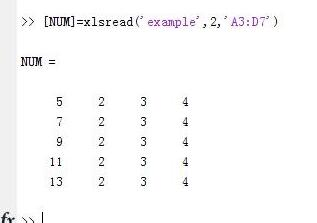快来学习学习Matlab读取excel文件里数据的操作流程吧，一定会帮到大家的。

展开全文• 读取多个excel表中多个sheet中的数据 数据分为train和test，train有12个excel表格，test有4个excel表格，每一个表格中工作薄的个数不一样，每个工作薄的数据行列数也不一定相同，经过尝试，MATLAB语句更成熟一些，...
读取多个excel表中多个sheet中的数据
数据分为train和test，train有12个excel表格，test有4个excel表格，每一个表格中工作薄的个数不一样，每个工作薄的数据行列数也不一定相同，经过尝试，MATLAB语句更成熟一些，Python语句与库有关系，没有MATLAB那么统一，但Python的确更人性化。 读取所有表格中所有sheet中的数据，单独储存到csv文件中。程序只放了test文件的数据读取。
MATLAB程序
files = dir('*.xlsx');
files_train = files(5:16,:);
files_test = files(1:4,:);
files_exchange = files_train;
files_train([2,3,4:12]) = files_exchange([11,12,2:10]);
clear files_exchange;
%因为不清楚每个sheet中数据size，这里设置了最大列数2500，
%经过测试，train最大是有1896列，test是有1910列。将数据储存在矩阵中，
%列数必须相同，选择手动补充零，这一点不如Python，Python可以自动补充'NAN'
len_max = 2500;
A = [];
B = [];
tic
for j = 1:length(files_test)
filenme = files_test(j).name;
[Type, Sheet]=xlsfinfo(filenme);
%sheet 返回文件中每个电子表格的名称
for i = 1:length(Sheet)
% i_1 = 12*(i-1)+1;
% i_2 = 12*i;
len = length(data);
data = [data, zeros(12, len_max-len)];
B = [B; data];
end
end
%删除多余零列
for k = 2500 :-1: 1881
no_zero = max(max(abs(B(:, k))));
if no_zero == 0
B(:,k) = [];
else
break;
end
end
waitbar(0.5);
writematrix(B, 'TestAll.csv');
toc

Python程序
import pandas as pd
import xlrd
import os
from pandas import DataFrame
import datetime, time

def TestDeal(files_test)
for fileT in files_test:
wb_T = xlrd.open_workbook(fileT)
sheets_T = wb_T.sheet_names()
sheets_test.append(sheets_T)
dataTest = DataFrame()
for sheet in range(len(sheets_T)):
index=False, encoding='utf8')
dataTest = dataTest.append(df_test, ignore_index=True)
print("Saving the teat data...")
return dataTest

starttime = datetime.datetime.now()
#windows是用\，而linux是用/，windows根目录是盘符C:\，而linux根目录是/
#inputdir 打开数据所在文件夹
#files = os.listdir(inputdir)
# 获得文件夹下的所有Excel文件
files = [x for x in os.listdir() if x.endswith(".xlsx")]
files_train = [files,files,files,files,files,files,files,\
files,files,files,files,files]
files_test = [files,files,files,files]

sheets_test, sheets_all = [], []
max_test, max_train = [], []
size_test, size_train = [], []
# read data and deal data
dataTest= TestDeal(files_test)
dataTrain= TrainDeal(files_train)

endtime = datetime.datetime.now()
print (endtime - starttime).seconds

# save data
start = time.time()   #windos 用clock
max_data = max_test.append(max_train)
# 创建pdDF格式数据，用于储存csv格式数据
data_save_train = DataFrame(dataTrain)
data_save_test = DataFrame(dataTest)
end = time.time()
print('runing time:%f', end-start)

展开全文python
最近在用matlab处理采集到的数据，这些数据都是按照一定的格式存入excel文件中的，每天对应产生一个文件。这些表格数量较多，所以通过matlab进行批量读取和处理。
num = xlsread(filename,sheet,xlRange)
通过这条函数可以获取指定文件指定表的指定范围的数值。为了能够循环读取，可以构造filename，读取多个文件。
%% 获得指标数据

yue = 7; %
A=[];
B=[];
num=[31,28,31,30,31,30,31,31,30,31,30,31];

xlRange =[ {'S2'},{'B3'},{'l26'},{'j28'},{'q26'}];
%
tic
for xx=1:1:length(xlRange);%5个数据
A=[];
for mon=1:1:yue;
for day=1:1:num(mon);
filename=['2018年',num2str(mon),'月', num2str(day),'日指标.xls']
sheet = '日报';
if(isempty(subsetA))
subsetA=NaN;
end
A=[A;subsetA];
end
end
B=[B A];
end
toc
解决了需要从多个表里找数据的问题，另外可以通过下面的方法读取表格的文本数据
[num,txt,raw] = xlsread(___)
在使用时发现这样读取文件特别费时，为了能够快速处理，打算将所有的文件读入内存，在内存里查找需要的数据
clear
clc

yue = 7; %
num=[31,28,31,30,31,30,31,31,30,31,30,31];
sheet = {'0点班 ','8点班','16点班','日报'};
BO=cell(2,length(sheet));
tic
for xx=1:1:length(sheet);%
nu=[];tx=[];id=0;nid=0;tid=0;
for mon=1:1:yue;
for day=1:1:num(mon);
filename=['2018年',num2str(mon),'月', num2str(day),'日指标.xls']
[len1 sz1]=size(nu);
[len2 sz2]=size(number);
len=len2-len1;
if(len>0)
nu=[nu;NaN(len,sz1)];
end
if(len<0)
number=[number;NaN(-len,sz2)];
end
nu=[nu number];%tx=[tx txt];%向右
id=id+1;[n nid(id+1)]=size(nu);[n tid(id+1)]=size(tx);
end
end
tid(id+1)=[]; nid(id+1)=[];

BO{1,xx}=nu;BO{2,xx}=nid;

end
toc
效果不错，读取文件之后速度飞快。解决目前处理数据的数据获取难题。
展开全文xlsread
• Matlab读取Excel各个子表的数据进行拟合 前言 现有一Excel文件，里面存有很个子表，每子表格式一样，但是数据不一样，现要对每子表中的好多行数据进行多项式拟合，并将拟合好的结果保存回Excel中各个子...
Matlab读取Excel各个子表的数据进行拟合
前言
现有一个Excel文件，里面存有很多个子表，每个子表格式一样，但是数据不一样，现要对每个子表中的好多行数据进行多项式拟合，并将拟合好的结果保存回Excel中各个子表的指定位置。这本来是可以通过人工将所要拟合的数据放到matlab下一次一次拟合再将结果人工保存到Excel中，但是这样太耗时，所以现在想通过Matlab自动读取Excel数据，拟合完成再将结果自动写入Excel中。
电脑环境：
1、Windows10教育版 64位 1803版本（操作系统版本：17134.165）  2、Matlab R2014a（8.3.0.532）64位
Matlab 代码实现
clc;clear;
y=[-65 -64 -63 -62 -61 -60 -59 -58 -57 -56 -55 -54 -53 -52 -51 -50 -49 -48 -47 -46 -45 -44 -43 -42 -41 -40 -39 -38 -37 -36 -35 -34 -33 -32 -31 -30];
filename = 'SignalStrengData_7to13.xlsx';%表格文件名
[status,sheets,xlFormat] = xlsfinfo(filename);%读取excel文件的信息，其中sheets为元胞类型，为excel中每张子表的名字
for sheet = 1:7%excel中的子表序号循环变量
folder = sheets{sheet};%当前子表的文件夹的名字,这里必须用{}来提取cell中的数据，不能用[]来提取
%     fprintf(folder)%testcode
mkdir (folder) %新建当前子表的文件夹，用于保存图片
for i=16:27%表格中的行循环次数
if isempty(x)%判断是否从Excel表格中读取到数据，若读取到的数据为空，则跳出该次循环
break;
end
xishu=polyfit(x,y,3);%三次多项式插值拟合得到系数
xishu2 = roundn(xishu, -2);%将系数只保留2位小数
result_xishu = sprintf('%.2f,%.2f,%.2f,%.2f', xishu2);%将系数格式化输出
fprintf ('\t\t所得系数为：')%打印系数查看一下
fprintf(result_xishu)
z=polyval(xishu2,x);%z为用拟合函数根据自变量算出来的值
h = figure(i);
plot(x,y,'r*',x,z,'b-');%绘图：拟合曲线和原始数据
set(h,'visible','off');
pictureName = sprintf('%s中D%d_AM%d的数据拟合结果', folder, i, i);%构建保存图片的文件名,注意：文件名中不能有冒号，所以此外将冒号改为了下划线
%         fprintf('\n')%testcode
%         fprintf(pictureName);%testcode
fprintf('\t正在保存图片到文件夹...')
filepath=pwd;           %保存当前工作目录
cd (folder) %把当前工作目录切换到指定文件夹
saveas(h, pictureName,'jpg');%保存绘图到指定文件夹
cd (filepath)
fprintf('\t已保存图片到(%s)文件夹中.',folder)
fprintf('\t正在写入数据到指定单元格...')
WriteRange = ['C',num2str(i)];%要写入的单元格
xlswrite(filename, {result_xishu}, sheet, WriteRange);%将数据写入单元格
fprintf('\t\t\t已将所得系数写入子表(%s)中%s单元格。\n', folder, WriteRange)
end
fprintf('\n子表(%s)  处理完成。\n\n',folder)
end
运行结果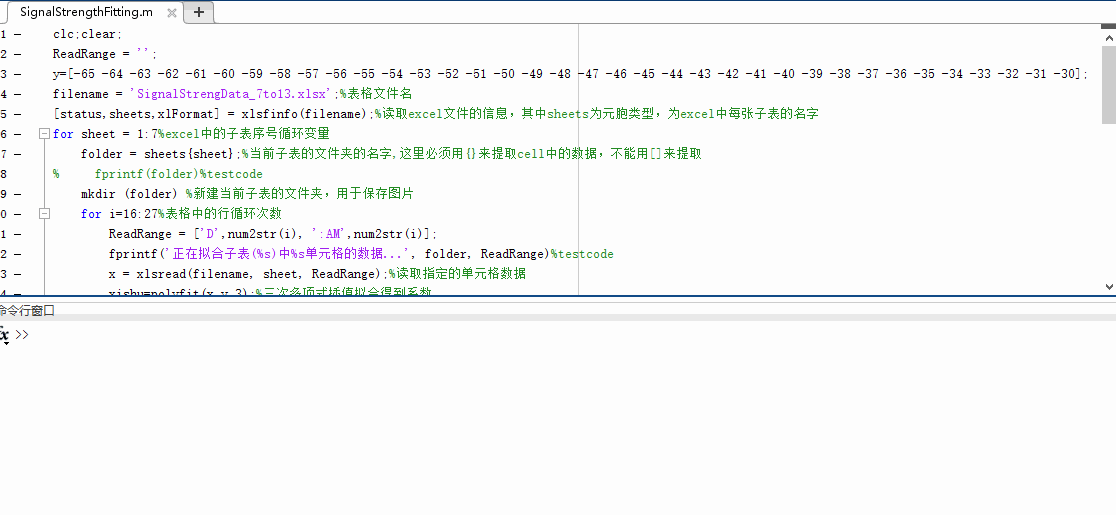生成拟合好的曲线的图片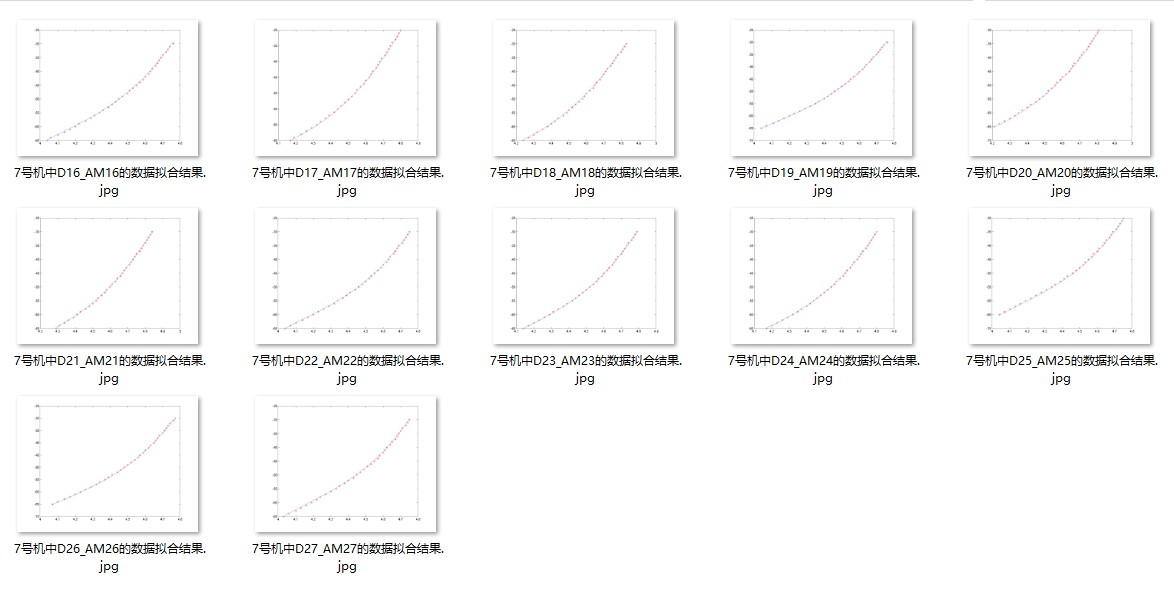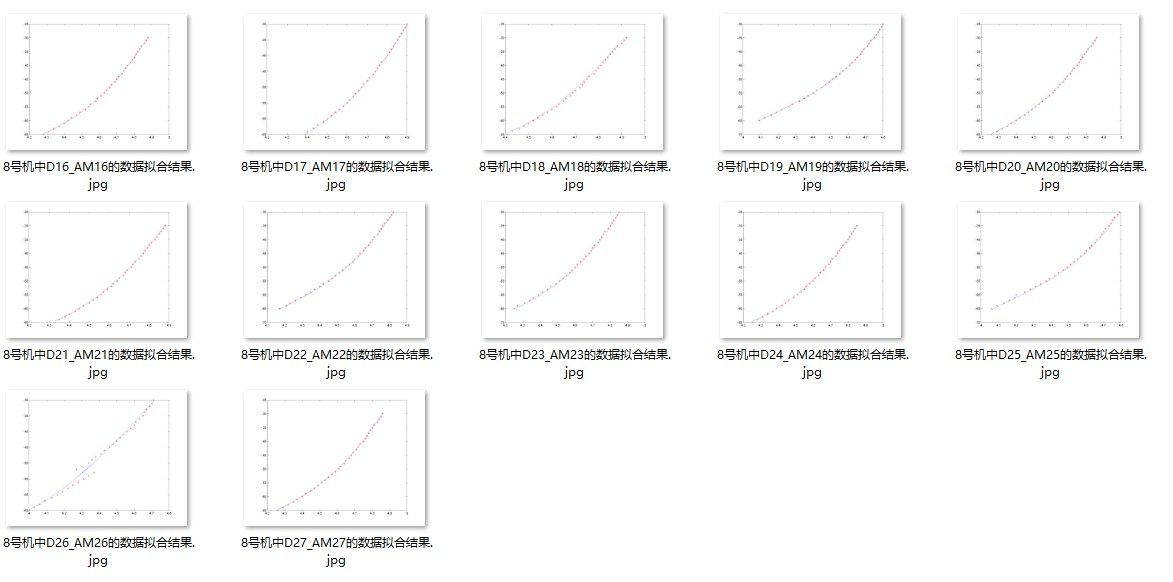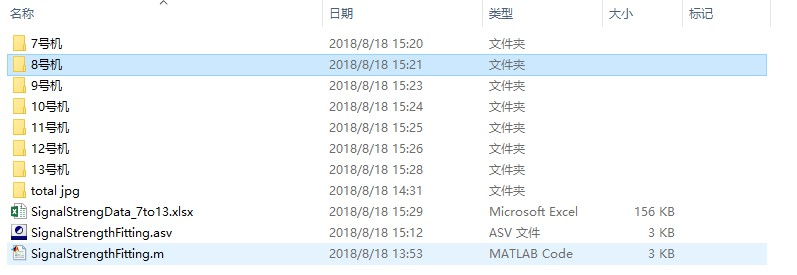总结
在其中遇到一些小问题，通过查资料都慢慢解决了，比如之前都没接触过matlab中的cell数据类型。需要注意的地方我都在代码中的注释中写好了。  因为考虑到可以在录数据到Excel中时，可能存在输入错误的情况，然后导致数据错误，以至于最后拟合出来的结果有问题，表现出来就是本该是平滑的曲线，结果有一个点两个点与其他点相差很大。为了解决这个问题，所以就将拟合得到的结果绘制成曲线图并进行保存，然后通过人为的方法来看各个曲线图是否有错误数据。
参考资料
1、曲线拟合 - 维基百科，自由的百科全书  2、插值 - 维基百科，自由的百科全书  3、matlab里面如何保留小数特定位数 - CSDN博客  4、MATLAB如何将数字数组转换成字符串？_百度知道  5、Matlab实现数字转换为字符串 - CSDN博客  6、matlab将字符/cell写入excel_FChChou_新浪博客  7、如何用matlab将一组字符串写入excel的一个格? – MATLAB中文论坛  8、Matlab连接字符串的方法无物新浪博客  9、用excel画拟合函数，导出公式_百度知道  10、excel相邻两列的数据自动求差值_百度知道  11、Excel 两个工作薄切换的快捷键是什么？-Excel基础应用-ExcelHome技术论坛 -  12、Excel2013如何将某数据范围的单元格以某种颜色突出显示_excel2013_office之家  13、Matlab实现保存图片到指定文件夹 - CSDN博客  14、matlab 保存figure中的图像 - CSDN博客  15、matlab批量保存图像至指定文件夹（revised） mkdir cd 等 - CSDN博客  16、matlab绘完图后可以不弹出figure而直接保存吗？ – MATLAB中文论坛  17、MATLAB中cell函数用法宝言新浪博客  18、matlab的cell数组玉琪星兆新浪博客  19、matlab 用循环画图并保存 如何不重叠 – MATLAB中文论坛
展开全文matlab 数据拟合
• MATLAB读取Excel，Txt文件中的数据 1.读取Excel中的数据 a = xlsreade('这里是文件路径D:\...\文件名.xls', 这里是sheet几,'A1:B5(这里是一矩形的范围)' )2.读取Txt中的数据 [name, type, x, y, answer] = ...数据
• 转自http://blog.chinaunix.net/uid-25067956-id-1743352.html在许多时候我们要借助于matlab读取excel的内容进行处理，以下是一种常用的处理方法office的表格文件也就是xls文件本质上就是一二维矩阵，二维矩阵是...
• 01功能介绍excel是我们常用的一款电子表格软件，其可以直观的展现我们收集的数据，并对其进行数据...而对于像作者一样，不懂vba但会matlab或python语言的使用者可以使用另一种方，即将excel的数据读入另外的编程软件...
• 一个excel表格中可能有一个Sheet或多个Sheet，虽然在MATLAB中只需要加些代码即可轻松实现，但是为了帮助各位更容易理解。因此，分为两部分讲解：1）excel表格中只有一个Sheet；2）excel表格中只有多个Sheet。 一 ...
• 我有很多excel,每个excel有若干个sheet,不同excel的sheet名不一样个数也不一样，我需要把每个sheet的mesh那一列数据提取出来，进行运算，最后把数据进行保存。运算的代码已经有了。 我在python上能做出来，但是计算...
• ## matlab对Excel表读取数据

万次阅读 多人点赞 2019-07-09 23:16:25
• 作者简介本文作者：FIFA少侠 本文由作者发布于技术邻，转载需要作者授权。 FIFA少侠，熟悉电力电力设备的有限元分析。... 一、MatlabExcel的交互1、引述：数学建模题目常会将数据以附件形式存放在Exc...
• matlabexcel里的sheet重命名； matlabExcel工作表重命名； 使用matlabexcel合并单元格； matlab导出excel时合并单元格；
• matlab 如何批量打开一个Excel中多个sheet表格同一列数组，并输入到新建Excel文件中VBA实现，请发送表格和说明到邮箱，点名字可查。matlab怎么读取excel中所有sheet的数据xlsinfo确定有多少个sheet[Type Sheet ...
• matlab读取多个数据文件合成一张图 具体来说就是将一个文件夹里的多个csv文件用matlab读取后再绘制成一张图 % file=dir('E:\0817\*.csv'); % 返回data文件夹下的文件列表 [f, p] = uigetfile({'*.csv', 'Data Files...
• matlab读取txt/excel文件为矩阵，并进行画图的方法 1. matlab准备 如果没有matlab的话，只需要到学校网站上下载，按照步骤就可以安装。THU的下载地址如下： THU matlab2021a下载地址 2. matlab读取txt文件以及excel...存储
• 首先，我的声明一下，我不是专门学习matlab的，我是学隧道工程的，所以我不专业，我主要学习的是matlab中一些智能算法的y回归预测，其他的没有学习，我只是想把自己所学的东西写一写，以便今后自己的学习，所以大家...office
• ## MATLAB如何读取excel文件中的数据？

万次阅读 多人点赞 2016-11-19 15:26:25
• filename是单引号括起来的带路径的文件名，函数直接读取filename所指文件的sheet1中的数据区域存储到双精度矩阵num中；其中，数据区域的选取规则是：对表格前几含有非数值的行（列）直接忽略，不算入数据区域；...
• %%%%%%%%%%%%%%%%%%%%%%%%%%%%%%%%%%%%%%%%%%%%%%%%%%%%%%%%%%%%%%...% 将实验过程中所保存的所有.txt文件,按照对应子实验,依次读取并保存到同一.xls/.xlsx文件的不同工作表内【以方便后续的统一处理】 %************...

# matlab读取excel多个sheetmatlab 订阅